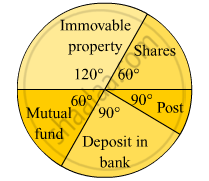# The Annual Investments of a Family Are Shown in the Adjacent Pie Diagram. Answer the Following Questions Based on It.(1) If the Investment in Shares is Rs 2000/, Find the Total Investment. - Algebra

The annual investments of a family are shown in the adjacent pie diagram. Answer the following questions based on it.
(1) If the investment in shares is Rs 2000/, find the total investment.
(2) How much amount is deposited in bank ?
(3) How much more money is invested in immovable property than in mutual fund ?
(4) How much amount is invested in post ?#### Solution

(1)
Money invested in shares = Rs 2000
$\Rightarrow \frac{60^\circ}{360^\circ} \times \text{ Total investment }= 2000$
$\Rightarrow\text{ Total investment }= \frac{360^\circ}{60^\circ} \times 2000$
$= Rs 12000$
(2)
Money invested in bank​

$= \frac{90^\circ}{360^\circ} \times\text{ Total investment }$
$= \frac{1}{4} \times 12000$
= Rs 3000
(3)
Difference in the central angle between immovable property and mutual fund = 120º − 60º
= 60º
Therefore, required mooney
$= \frac{60^\circ}{360^\circ} \times\text{ Total investment }$
$= \frac{1}{6} \times 12000$
= Rs 2000
(4)
Money invested in post​
$= \frac{30^\circ}{360^\circ} \times\text{ Total investment }$
$= \frac{1}{12} \times 12000$

= Rs 1000

Concept: Concept of Pie Graph (Or a Circle-graph)
Is there an error in this question or solution?